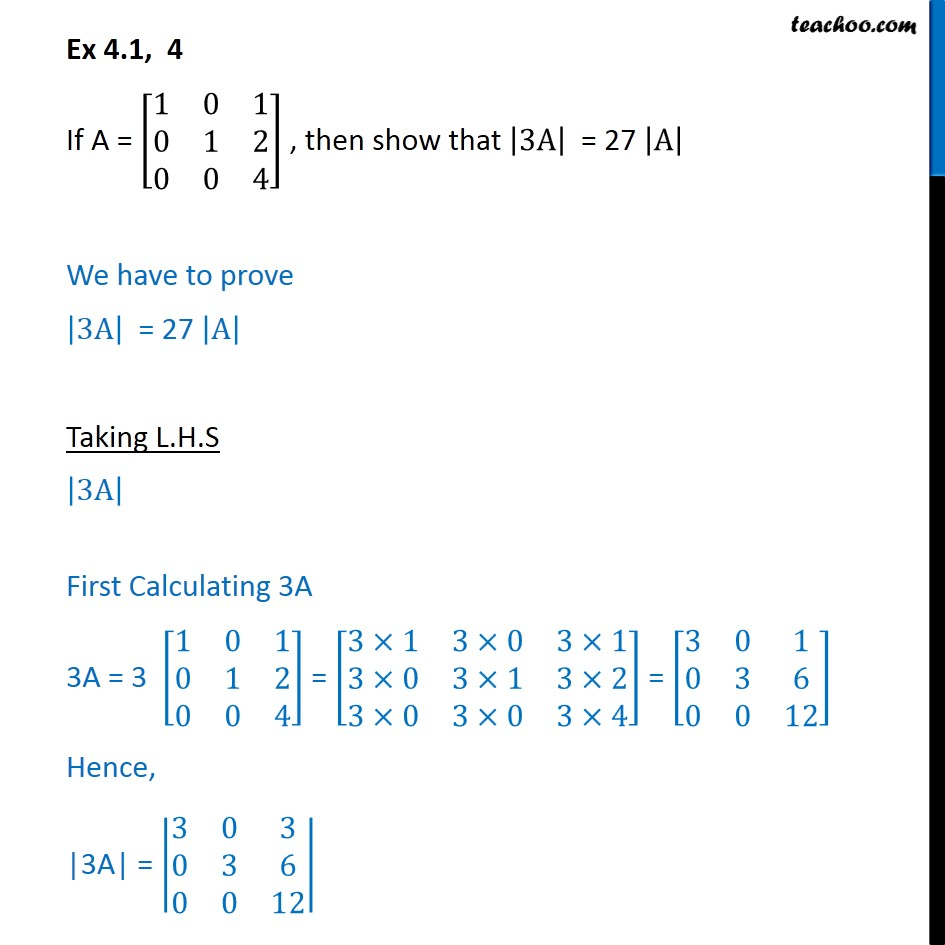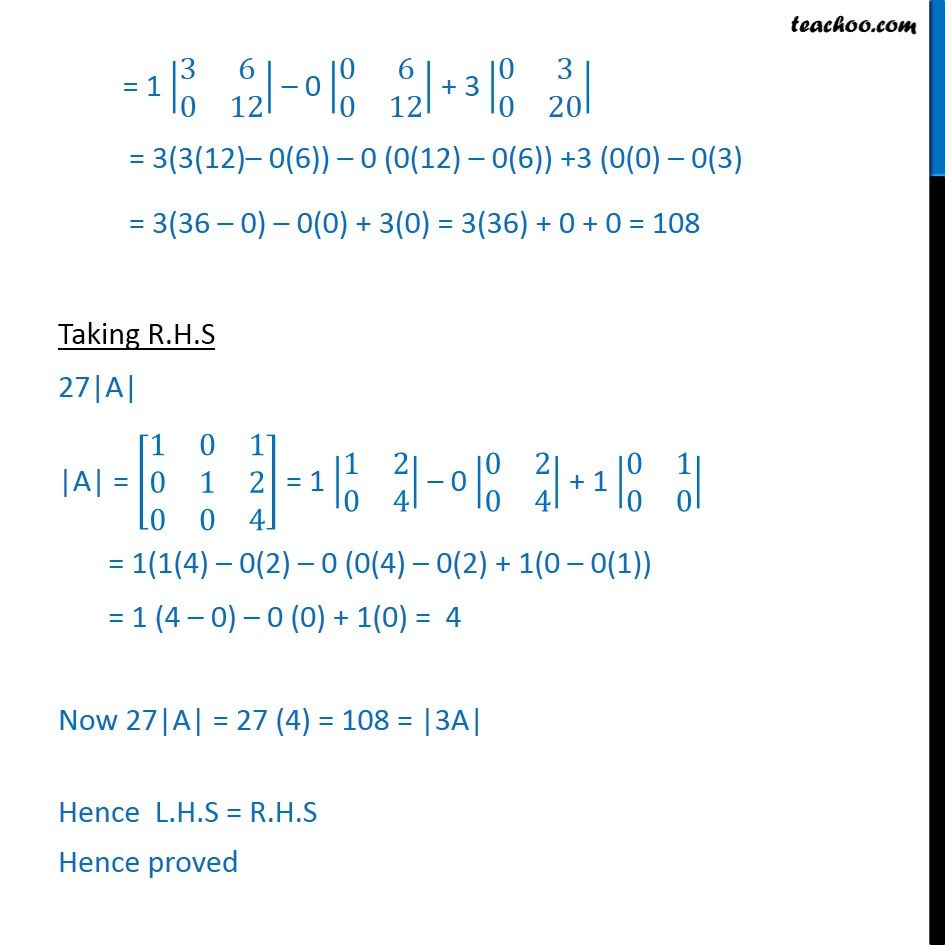1. Chapter 4 Class 12 Determinants
2. Concept wise
3. Evalute determinant of a 3x3 matrix

Transcript

Ex 4.1, 4 If A = 1 0 1 0 1 2 0 0 4 , then show that 3A = 27 A We have to prove 3A = 27 A Taking L.H.S 3A First Calculating 3A 3A = 3 1 0 1 0 1 2 0 0 4 = 3 1 3 0 3 1 3 0 3 1 3 2 3 0 3 0 3 4 = 3 0 1 0 3 6 0 0 12 Hence, |3A| = 3 0 3 0 3 6 0 0 12 = 1 3 6 0 12 0 0 6 0 12 + 3 0 3 0 20 = 3(3(12) 0(6)) 0 (0(12) 0(6)) +3 (0(0) 0(3) = 3(36 0) 0(0) + 3(0) = 3(36) + 0 + 0 = 108 Taking R.H.S 27|A| |A| = 1 0 1 0 1 2 0 0 4 = 1 1 2 0 4 0 0 2 0 4 + 1 0 1 0 0 = 1(1(4) 0(2) 0 (0(4) 0(2) + 1(0 0(1)) = 1 (4 0) 0 (0) + 1(0) = 4 Now 27|A| = 27 (4) = 108 = |3A| Hence L.H.S = R.H.S Hence proved

Evalute determinant of a 3x3 matrix